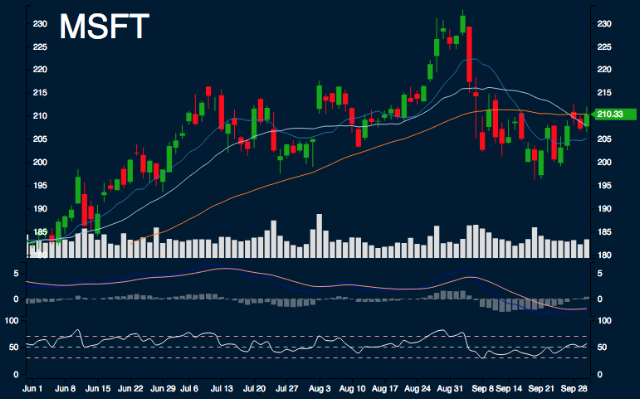#MSFT Report

Sample Size: 7; Percent Error: 10%

## Current Trend Performance

MSFT is -10.14% from monthly high, 4.73% from monthly low, 2.51% from 10 day simple moving average, 1.18% from 20 day simple moving average, and -0.04% from 50 day simple moving average.

## Forecast Ranges

Day 1:
The highs are 212.13, the lows are 205.34, and the median is 208.74. 33.33% to decrease between -2.68% - 0%. 66.67% to increase between 0% - 2.68%.
Day 2:
The highs are 214.77, the lows are 203.51, and the median is 209.14. 14.29% to decrease between -5.36% - -2.68%. 42.86% to decrease between -2.68% - 0%. 42.86% to increase between 0% - 2.68%.
Day 3:
The highs are 215.53, the lows are 200.74, and the median is 208.13. 14.29% to decrease between -5.36% - -2.68%. 42.86% to decrease between -2.68% - 0%. 42.86% to increase between 0% - 2.68%.
Day 4:
The highs are 217.64, the lows are 201.66, and the median is 209.65. 28.57% to decrease between -5.36% - -2.68%. 42.86% to decrease between -2.68% - 0%. 14.29% to increase between 0% - 2.68%. 14.29% to increase between 2.68% - 5.36%.
Day 5:
The highs are 214.23, the lows are 202.1, and the median is 208.16. 28.57% to decrease between -5.36% - -2.68%. 42.86% to decrease between -2.68% - 0%. 28.57% to increase between 0% - 2.68%.
Day 6:
The highs are 210.82, the lows are 201.04, and the median is 205.93. 14.29% to decrease between -5.36% - -2.68%. 57.14% to decrease between -2.68% - 0%. 28.57% to increase between 0% - 2.68%.
Day 7:
The highs are 210.33, the lows are 201.22, and the median is 205.78. 28.57% to decrease between -5.36% - -2.68%. 71.43% to decrease between -2.68% - 0%.

## Summary

SMA: Simple Moving Average

In next 5 days, there is possible support range between 204.69 and 206.3. There is possible resistance range between 211.94 and 215.97. Areas of uncertainty are beyond 203.12 and beyond 220.1. Minimum movement range is 2.68%.

Research conducted with Nforecast.

This is not a recommendation to buy, sell, or hold. This research is for educational, informational, and entertainment purposes. You should not construe any data or information presented by members on this blog or Nforecast LLC as investment, tax, legal, financial or other advice.